## Concepts and Definitions

Does your income put you in the upper half of all income earners? You can find the answer by comparing your income with the median income.

But what is the median? It is the “middle value” in a group after all observations are arranged in ascending order, i.e. from smallest to largest. At least half of the observations are equal to or smaller than the median, and at least half of the measurements are equal to or greater than the median. The median divides the bottom half of observations from the upper half.

How to find the median?

Step 1: Given a set of data (e.g. wages), arrange the numbers in ascending order i.e. from smallest to largest.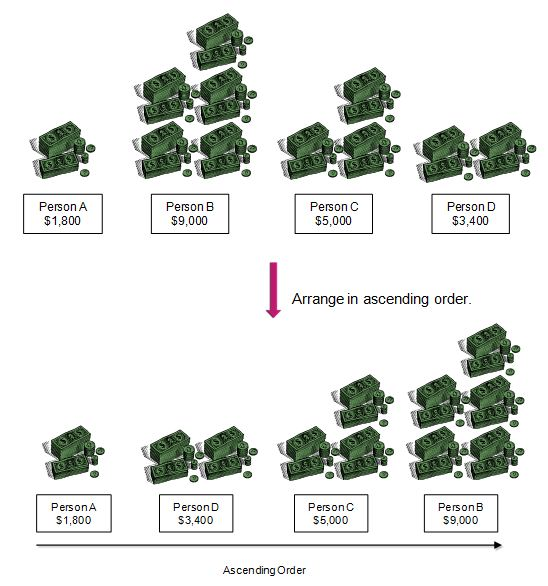Step 2:​ ​ ​ If the number of observations is odd, the number in the middle of the list is the median. This can be found by taking the value of the (n+1)/2 -th term, where n is the number of observations.​ ​ ​ Else, if the number of observations is even, then the median is the simple average of the middle two numbers. In calculation, the median is the simple average of the n/2 -th and the (n/2 + 1) -th terms.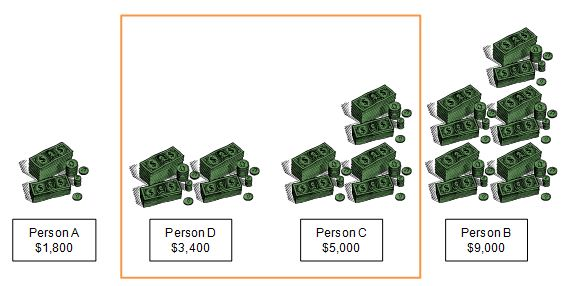As there are four observations (i.e. even number), the median is the simple average of the 2nd and 3rd lowest persons’ wages.  Therefore,
Median = ½ (Person D’s wage + Person C’s wage)
= ½ (3,400 + 5,000) = \$4,200.

Example 1 : Who’s In the Middle? (Odd Number of Observations)

To compare its employees’ age profile against that of other companies in the industry, your company, Middle World Co. has asked you to calculate the median age of your fellow workers.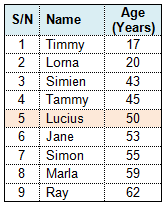What is the median age of these nine workers?

Solution: Since there are an odd number of observations and the ages are arranged from youngest to oldest (i.e. in ascending order), the median age is the age of the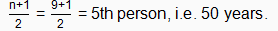Note that if Middle World does not hire new workers and no workers leave, Lucius’s age will always be the median age, no matter how many years have passed.

Example 2 : Wage Comparisons (Even Number of Observations)

Suppose we have the monthly wages of 10 employees of a company. How would you find the median wage?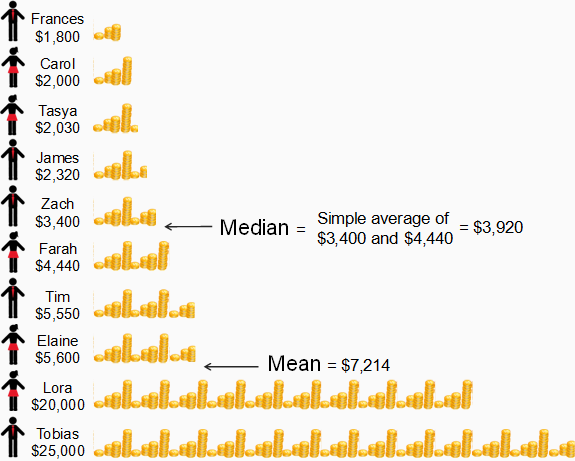Solution: Notice that we have arranged their wages above from lowest to highest. This ranking will help us to determine the median. Using the method introduced earlier, the median is computed by taking the simple average of the (n/2)-th = (10/2)-th = 5th and (n/2 + 1)-th = (10/2+1)-th = 6th observations.

Therefore, the median is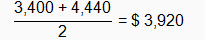How does the median differ from the mean?

Note that the mean is \$7,214, which is very much higher than the median value. This difference in values demonstrates that the median is unaffected by a small number of extreme values. (The extreme values here, or outliers, are \$20,000 and \$25,000.)